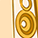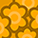cancel
Showing results for
Did you mean:New Contributor

## How to calculate the molarity of a buffer?

I have given the concentration of L-Histidine (FW: 155.155g/mol) at 0.24g/L and concentration of Histidine HCl Monohydrate (FW:169.6g/mol) at 3.87g/L. How do I calculate the molarity of buffer?

2 RepliesNew Contributor III

## Re: How to calculate the molarity of a buffer?

To calculate the molarity (M) of a buffer solution, you need to know the following information:

1. The amount of the acid (HA) and its molar mass (MHA).
2. The amount of the conjugate base (A-) and its molar mass (MA-).
3. The volume of the solution (in liters, L).

The formula for calculating the molarity of a buffer solution is:

M = (moles of buffer component) / (volume of solution in L)

To calculate the moles of each component of the buffer, you can use the following formulas:

For the acid (HA): Moles of HA = (mass of HA) / (molar mass of HA)

For the conjugate base (A-):

EgyptNew Contributor

## Re: How to calculate the molarity of a buffer?

To calculate the molarity of a buffer solution, you need to follow these steps:

Convert mass to moles:

For L-Histidine:

moles of L-Histidine= molar massmass/massmolar massmoles of L-Histidine

moles of L-Histidine=0.24g/155.155g/mol

For Histidine HCl Monohydrate:

moles of Histidine HCl Monohydrate=mass / molar mass

moles of Histidine HCl Monohydrate=3.87 g/ 169.6 g/mol

Calculate total moles of solute:

Total moles of solute=moles of L-Histidine+moles of Histidine HCl Monohydrate

Calculate volume of solution in liters:

• If concentrations are given in g/L, the volume in liters can be directly used as 1 L.
• If concentrations are given in other units, convert them to g/L.

Calculate Molarity: Molarity=Total moles of solute / Volume of solution (in liters)

Just plug in the values into the formula to get the molarity of the buffer solution.